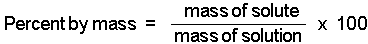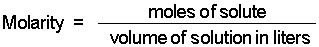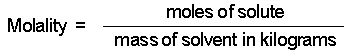Concentrations of Solutions

There are a number of ways to express the relative amounts of solute and solvent in a solution. This page describes calculations for four different units used to express concentration:

Percent Composition (by mass)

We can consider percent by mass (or weight percent, as it is sometimes called) in two ways:

• The parts of solute per 100 parts of solution.
• The fraction of a solute in a solution multiplied by 100.
We need two pieces of information to calculate the percent by mass of a solute in a solution:
• The mass of the solute in the solution.
• The mass of the solution.

Use the following equation to calculate percent by mass:Top
Molarity

Molarity tells us the number of moles of solute in exactly one liter of a solution. (Note that molarity is spelled with an "r" and is represented by a capital M.)

We need two pieces of information to calculate the molarity of a solute in a solution:

• The moles of solute present in the solution.
• The volume of solution (in liters) containing the solute.

To calculate molarity we use the equation:Top
Molality

Molality, m, tells us the number of moles of solute dissolved in exactly one kilogram of solvent. (Note that molality is spelled with two "l"'s and represented by a lower case m.)

We need two pieces of information to calculate the molality of a solute in a solution:

• The moles of solute present in the solution.
• The mass of solvent (in kilograms) in the solution.

To calculate molality we use the equation:Top
Mole Fraction

The mole fraction, X, of a component in a solution is the ratio of the number of moles of that component to the total number of moles of all components in the solution.

To calculate mole fraction, we need to know:

• The number of moles of each component present in the solution.

The mole fraction of A, XA, in a solution consisting of A, B, C, ... is calculated using the equation:To calculate the mole fraction of B, XB, use:Top